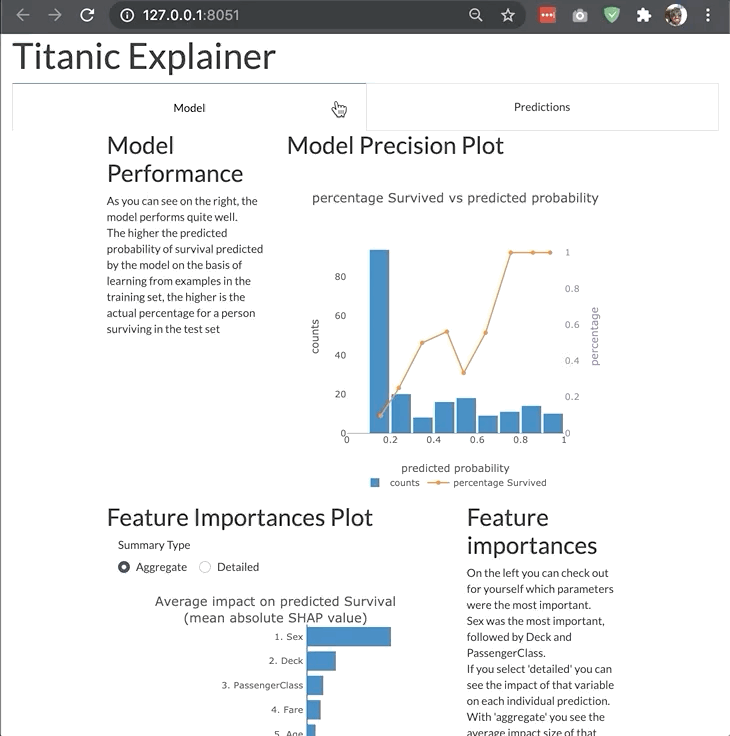The dashboard is highly modular and customizable so that you can adjust it your own needs and project. You can switch off tabs, control the features of invidual components in the dashboard, or even build your entire custom dashboard layout like the example below:## Changing bootstrap theme¶

You can change the bootstrap theme by passing a link to the appropriate css file. You can use the convenient themes module of dash_bootstrap_components to generate the css url for you:

```import dash_bootstrap_components as dbc

ExplainerDashboard(explainer, bootstrap=dbc.themes.FLATLY).run()
```

See the dbc themes documentation for the different themes that are supported.

## Switching off tabs¶

You can switch off individual tabs using boolean flags, e.g.:

```ExplainerDashboard(explainer,
importances=False,
model_summary=True,
contributions=True,
whatif=True,
shap_dependence=True,
shap_interaction=False,
decision_trees=True)
```

## Hiding components¶

You can also hide individual components on the various tabs:

```ExplainerDashboard(explainer,
# importances tab:
hide_importances=True,
# classification stats tab:
hide_globalcutoff=True, hide_modelsummary=True,
hide_confusionmatrix=True, hide_precision=True,
hide_classification=True, hide_rocauc=True,
hide_prauc=True, hide_liftcurve=True, hide_cumprecision=True,
# regression stats tab:
# hide_modelsummary=True,
hide_predsvsactual=True, hide_residuals=True,
hide_regvscol=True,
# individual predictions tab:
hide_predindexselector=True, hide_predictionsummary=True,
hide_contributiongraph=True, hide_pdp=True,
hide_contributiontable=True,
# whatif tab:
hide_whatifindexselector=True, hide_inputeditor=True,
hide_whatifcontribution=True, hide_whatifpdp=True,
# shap dependence tab:
hide_shapsummary=True, hide_shapdependence=True,
# shap interactions tab:
hide_interactionsummary=True, hide_interactiondependence=True,
# decisiontrees tab:
hide_treeindexselector=True, hide_treesgraph=True,
hide_treepathtable=True, hide_treepathgraph=True,
).run()
```

## Hiding toggles and dropdowns inside components¶

You can also hide individual toggles and dropdowns using **kwargs. However they are not individually targeted, so if you pass hide_cats=True then the group cats toggle will be hidden on every component that has one:

```ExplainerDashboard(explainer,
no_permutations=True, # do not show or calculate permutation importances
hide_popout=True, # hide the 'popout' button for each graph
hide_poweredby=True, # hide the 'powerered by: explainerdashboard' footer
hide_popout=True, # hide the 'popout' button from each graph
hide_depth=True, # hide the depth (no of features) dropdown
hide_sort=True, # hide sort type dropdown in contributions graph/table
hide_orientation=True, # hide orientation dropdown in contributions graph/table
hide_type=True, # hide shap/permutation toggle on ImportancesComponent
hide_dropna=True, # hide dropna toggle on pdp component
hide_sample=True, # hide sample size input on pdp component
hide_gridlines=True, # hide gridlines on pdp component
hide_gridpoints=True, # hide gridpoints input on pdp component
hide_cutoff=True, # hide cutoff selector on classification components
hide_percentage=True, # hide percentage toggle on classificaiton components
hide_log_x=True, # hide x-axis logs toggle on regression plots
hide_log_y=True, # hide y-axis logs toggle on regression plots
hide_ratio=True, # hide the residuals type dropdown
hide_points=True, # hide the show violin scatter markers toggle
hide_winsor=True, # hide the winsorize input
hide_wizard=True, # hide the wizard from the lift curve
)
```

## Setting default values¶

You can also set default values for the various dropdowns and toggles. All the components with their parameters can be found in the componentsdocumentation. Some examples of useful parameters to pass:

```ExplainerDashboard(explainer,
index='Rugg, Miss. Emily', # initial index to display
col='Fare', # initial feature in shap graphs
color_col='Age', # color feature in shap dependence graph
interact_col='Age', # interaction feature in shap interaction
higher_is_better=False, # flip green and red in contributions graph
depth=5, # only show top 5 features
sort = 'low-to-high', # sort features from lowest shap to highest in contributions graph/table
orientation='horizontal', # horizontal bars in contributions graph
cats_topx = 3, # show only the top 3 categories
cats_sort = 'shap', # sort categories by mean abs shap instead of 'freq' or 'alphabet'
pdp_col='Fare', # initial pdp feature
cutoff=0.8, # cutoff for classification plots
round=2 # round floats to 2 digits
show_metrics=['accuracy', 'f1', custom_metric] # only show certain metrics
plot_sample=1000, # only display a 1000 random markers in scatter plots
)
```

## Using custom metrics¶

By default the dashboard shows a number of metrics for classifiers (accuracy, etc) and regression models (R-squared, etc). You can control which metrics are shown and in what order by passing `show_metrics`:

```ExplainerDashboard(explainer, show_metrics=['accuracy', 'f1', 'recall']).run()
```

However you can also define custom metrics functions yourself as long as they take `y_true` and `y_pred` as parameters:

```def custom_metric(y_true, y_pred):
return np.mean(y_true)-np.mean(y_pred)

ExplainerDashboard(explainer, show_metrics=['accuracy', custom_metric]).run()
```

For `ClassifierExplainer`, `y_true` and `y_pred` will have already been calculated as an array of `1` and `0` depending on the `pos_label` and `cutoff` that was passed to `explainer.metrics()`. However, if you take `pos_label` and `cutoff` as parameters to the custom metric function, then you will get the unprocessed raw labels and pred_probas. So for example you could calculate a sum of cost function over the confusion matrix as a custom metric. Then the following metrics would all work and have the equivalent result:

```from sklearn.metrics import confusion_matrix

def cost_metric(y_true, y_pred):
cost_matrix = np.array([[10, -50], [-20, 10]])
cm = confusion_matrix(y_true, y_pred)
return (cost_matrix * cm).sum()

def cost_metric2(y_true, y_pred, cutoff):
return cost_metric(y_true, np.where(y_pred>cutoff, 1, 0))

def cost_metric3(y_true, y_pred, pos_label):
return cost_metric(np.where(y_true==pos_label, 1, 0), y_pred[:, pos_label])

def cost_metric4(y_true, y_pred, cutoff, pos_label):
return cost_metric(np.where(y_true==pos_label, 1, 0),
np.where(y_pred[:, pos_label] > cutoff, 1, 0))

explainer.metrics(show_metrics=[cost_metric, cost_metric2, cost_metric3, cost_metric4]).run()
```

Note

When storing an `ExplainerDashboard.to_yaml()` the custom metric functions will be stored to the `.yaml` file with a reference to their name and module. So when loading the dashboard `from_config()` you have to make sure the metric function can be found by the same name in the same module (which could be `__main__`), otherwise the dashboard will fail to load.